#### You may also like### Pent

The diagram shows a regular pentagon with sides of unit length. Find all the angles in the diagram. Prove that the quadrilateral shown in red is a rhombus.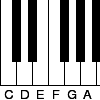### Tuning and Ratio

Why is the modern piano tuned using an equal tempered scale and what has this got to do with logarithms?### Rarity

Show that it is rare for a ratio of ratios to be rational.

# Another Triangle in a Triangle

##### Age 16 to 18 Challenge Level:

Sadaf from BSS in Pakistan found the relationship between then blue and green triangles: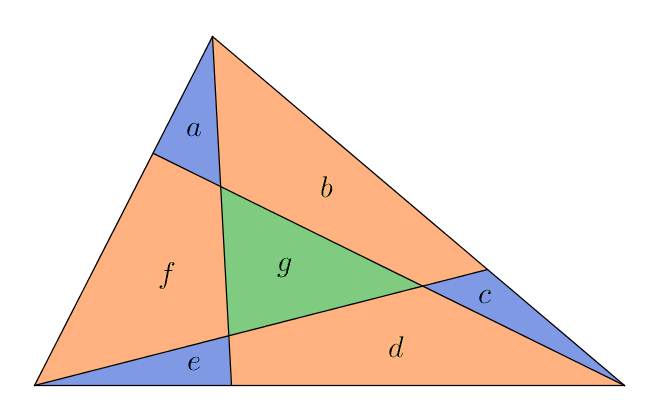$a+f+e$ to total $=1:3$
$a+b+c$ to total $=1:3$
$c+d+e$ to total $= 1:3$

using concept that ratio of areas of triangles with the same height is equal to ratio of their bases

(This idea can be seen in Triangle in a Triangle and in Areas and Ratios - breaking a triangle into two smaller triangles by dividing one of its sides in the ratio $a:b$, the areas of the two smaller triangles will also be in the ratio $a:b$)

Now three one thirds add up to a whole
$2a+b+2c+d+2e+f=$ one whole
this implies that
$a+c+e =g$

Reyna from Downside School used this same logic on many smaller triangles within the triangle. This is Reyna's work, with some additional diagrams added: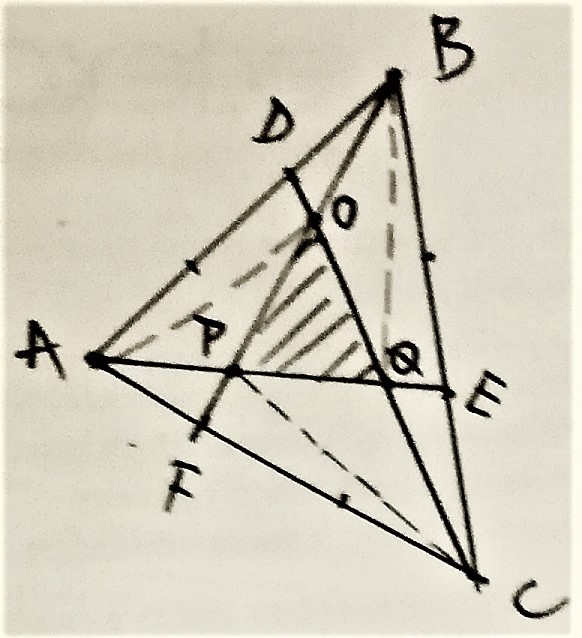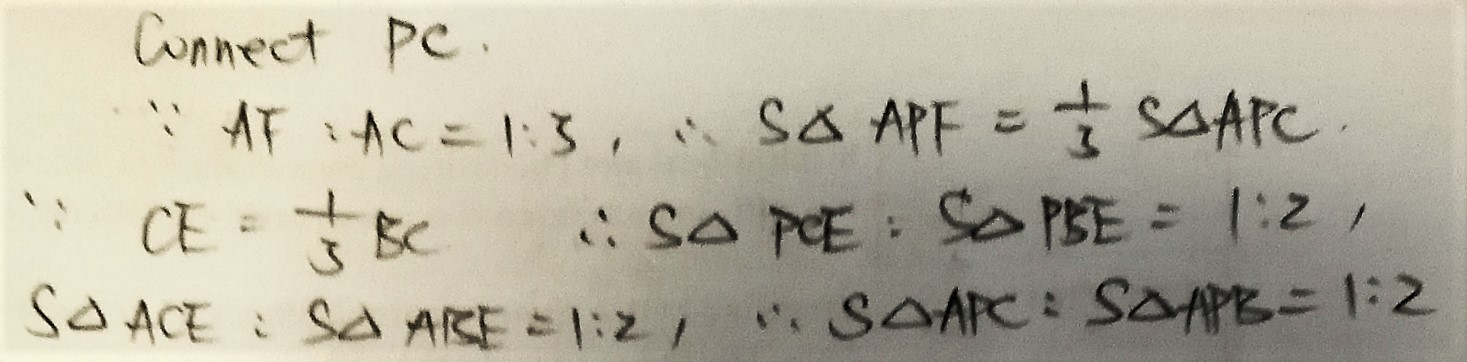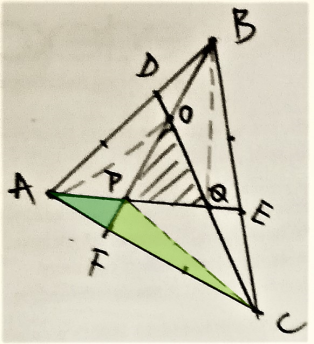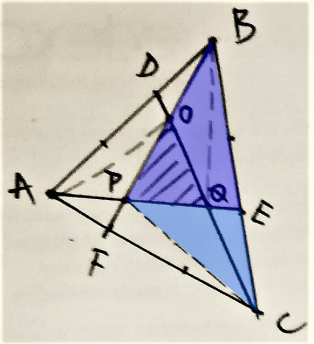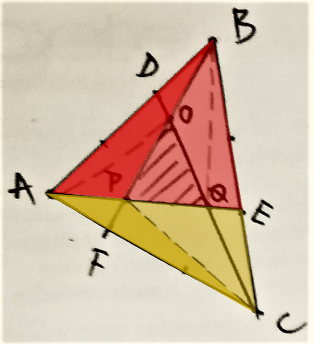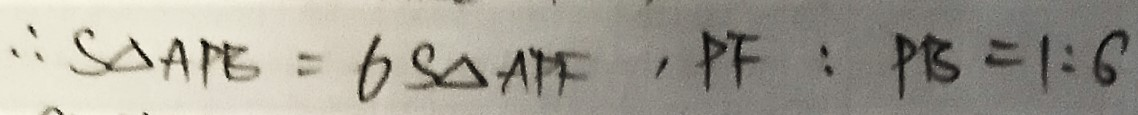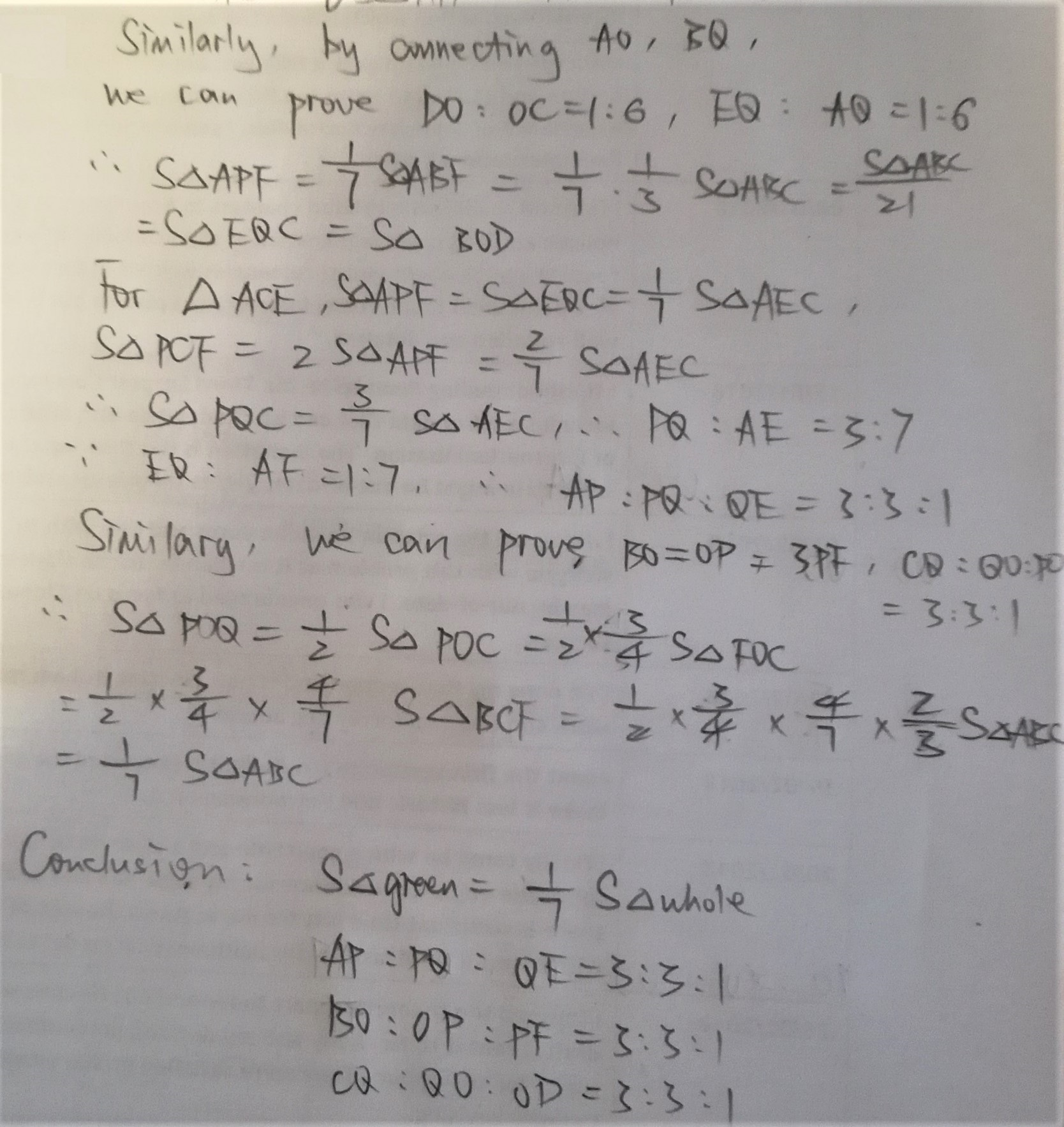Jamie from Bournemouth School in the UK considered a more general case where each side is divided into $n$ sections. Jamie then used vectors to find the area of the green triangle in this general case, and then used $n=3$. This is Jamie's work: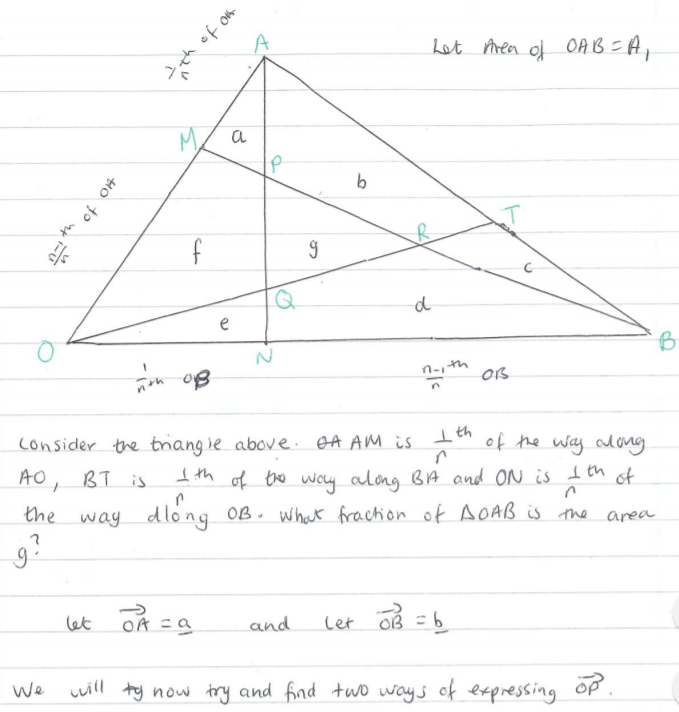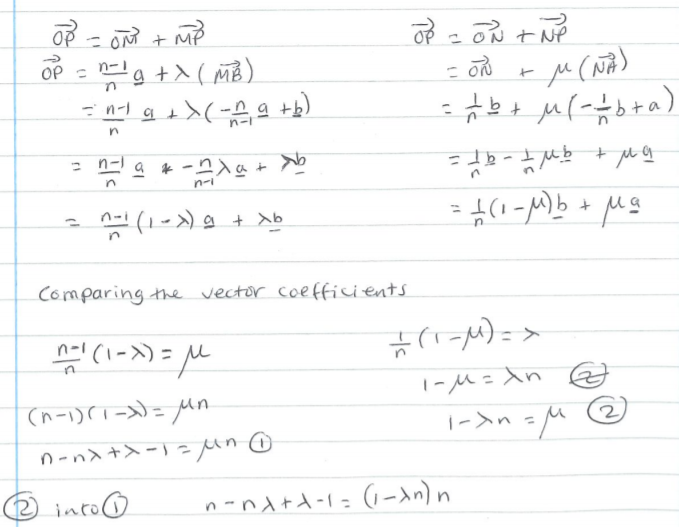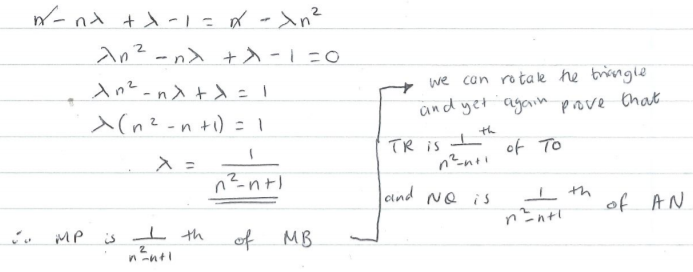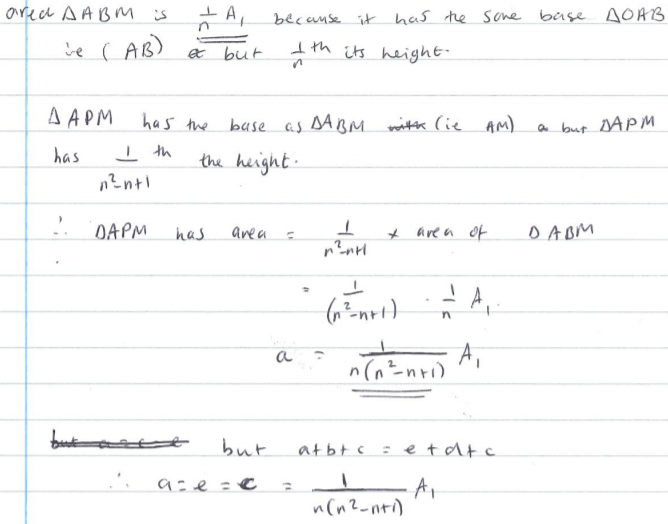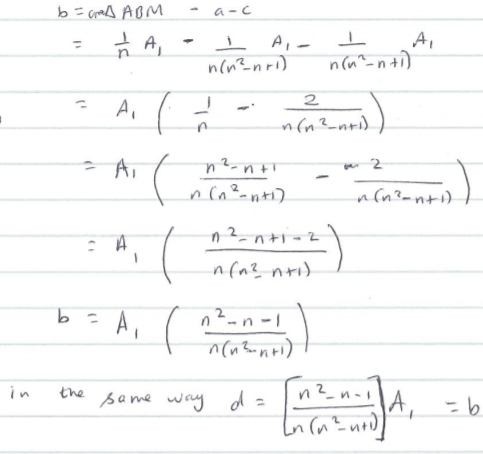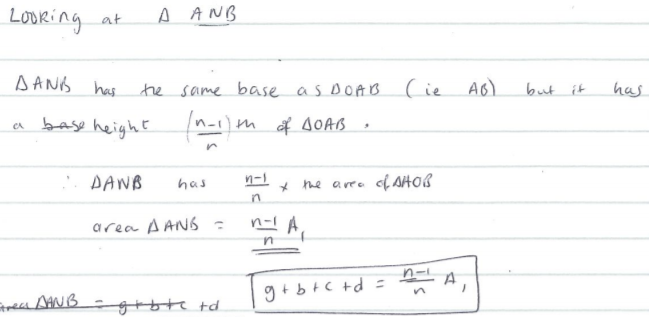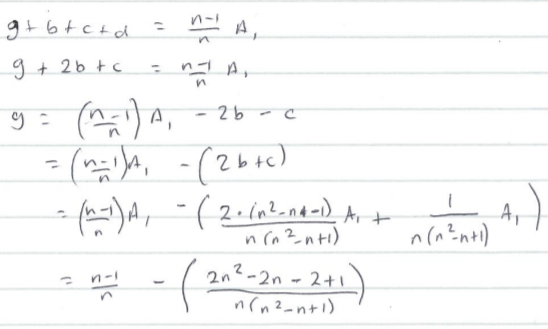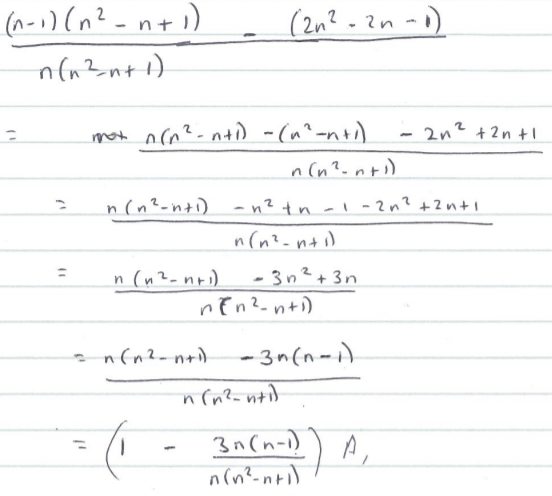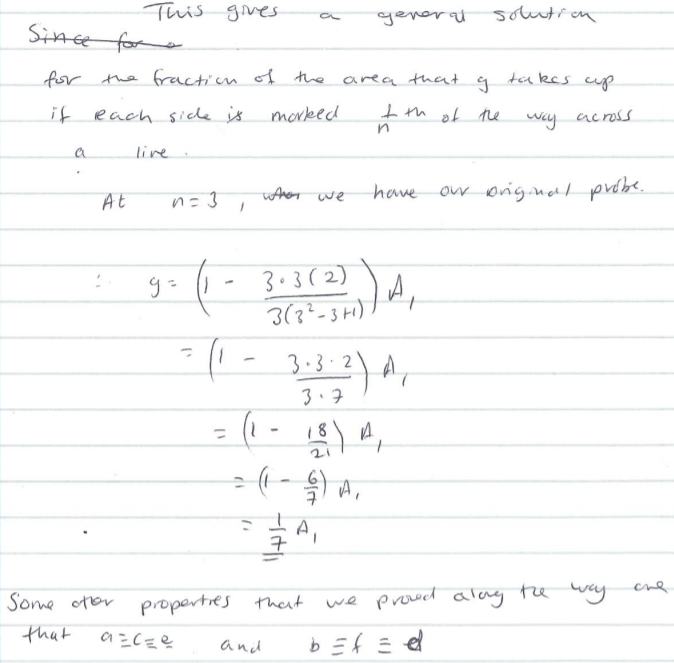Hoytem from Noodres college, Madras used coordinate geometry. This had a lot in common with Jamie's solution, but the coordinate geometry approach involves more algebra (even though Jamie was working in a more general case):
Attacking the problem with brute force will give results

Let the bottom left hand vertex of the largest triangle be $O$, the origin. Then, going anticlockwise around the triangle, let the other vertices be $A$ with coordinates $\left(a_x,0\right)$ then $B$ with coordinates $\left(b_x,b_y\right)$. Let $E$ be the point a third of the way along $BO$, with coordinates $\left(\frac23b_x, \frac23b_y\right)$, $D$ be the point on $OA$, with coordinates $\left(\frac13a_x,0\right)$ and $C$ be the point on $AB$, with coordinates $\left(\frac23a_x+\frac13b_x,\frac13b_y\right)$. These coordinates can be obtained by using the rules of similar triangles.

Finally, let the top vertex of the green triangle be $F$, with coordinates $\left(f_x,f_y\right)$ and, going clockwise around the triangle, let the other vertices be $G$, with coordinates $\left(g_x,g_y\right)$ then $H$, with coordinates $\left(h_x,h_y\right).$

In order to find the area of triangle, the following calculation can be done: $$A=\frac12(HF)(HG)\sin{F\hat{H}G}$$
First, the coordinates of $F$, $G$ and $H$ need to be found. (In terms of $a_x, b_x$ and $b_y$)

The equation of the line $BD$ is $$\frac{y-0}{x-\tfrac13a_x}=\frac{b_y-0}{b_x-\tfrac13a_x}\\ \Rightarrow y=\frac{3b_y}{3b_x-a_x}x-\frac{a_xb_y}{3b_x-a_x}$$
The equation of the line $EA$ is $$\frac{y-0}{x-a_x}=\frac{\tfrac{2}3b_y-0}{\tfrac23b_x-a_x}\\ \Rightarrow y=\frac{2b_y}{2b_x-3a_x}x-\frac{2a_xb_y}{2b_x-3a_x}$$
An equation can now be set up in $f_x$ (since the point $F$ is the intersection between the line $EA$ and the line $BD$): \begin{align}&\frac{3b_y}{3b_x-a_x}f_x-\frac{a_xb_y}{3b_x-a_x}=\frac{2b_y}{2b_x-3a_x}f_x-\frac{2a_xb_y}{2b_x-3a_x}\\ \Rightarrow & 3b_y\left(2b_x-3a_x\right)f_x-a_xb_y\left(2b_x-a_x\right)=2b_y\left(3b_x-a_x\right)f_x-2a_xb_y\left(3b_x-a_x\right)\\ \Rightarrow &\left(6b_xb_y-9a_xb_y\right)f_x-\left(6b_xb_y-2a_xb_y\right)f_x=a_xb_y\left(2b_x-3a_x\right)-2a_xb_y\left(3b_x-a_x\right)\\ \Rightarrow &\left(6b_xb_y-9a_xb_y-6b_xb_y+2a_xb_y\right)f_x=2a_xb_xb_y-3a_x^2b_y-6a_xb_xb_y+2a_x^2b_y\\ \Rightarrow&-7a_xb_yf_x=-a_xb_y\left(4b_x+a_x\right)\\ \Rightarrow &=f_x=\frac{a_x+4b_x}7\end{align}

We can now find $f_y$, since $\left(f_x,f_y\right)$ lies on the line $BD$ \begin{align}f_y&=\frac{3b_y}{3b_x-a_x}\left(f_x-\frac{a_x}3\right)\\ \Rightarrow f_y&=\frac{3b_y}{3b_x-a_x}\left(\frac{a_x+4b_x}7-\frac{a_x}3\right)\\ \Rightarrow f_y&=\frac{3b_y}{3b_x-a_x}\left(\frac{4\left(3b_x-a_x\right)}{21}\right)\\ \Rightarrow f_y&=\frac{4}{7}b_y\end{align}

The same process can be repeated to find the coordinates of $G$ and $H$, using the information that $G$ is the point of intersection between the lines $OC$ and $EA$ and $H$ is the point of intersection between the lines $OD$ and $BD$.

The equation of the line $OC$ is $$y=\frac{b_y}{2a_x+b_x}x$$
Using this information and the information above, the coordinates of $G$ are $$\left(\frac{4a_x+2b_x}7,\frac27b_y\right)$$
And the coordinates of $H$ are $$\left(\frac{2a_x+b_x}7,\frac17b_y\right)$$
Now, the length of $HF$ can be found. Let $HF_x=h_x-f_x$: $$\Rightarrow HF_x=\frac {2a_x+b_x-a_x-4b_x}{7}\\ \Rightarrow HF_x=\frac{a_x-3b_x}7$$
Similarly, let $HF_y=h_y-f_y$ \Rightarrow HF_y=\frac{b_y-4b_y}7\\ \Rightarrow HF_y=\frac{-3}{7}b_y\\ \begin{align} & HF=\sqrt{\left(HF_x\right)^2+\left(HF_y\right)^2}\\ \Rightarrow & HF = \frac{\sqrt{\left(a_x-3b_x\right)^2+\left(3b_y\right)^2}}7\\ \Rightarrow & HF = \frac{\sqrt{a_x^2-6a_xb_x+9b_x^2+9b_y^2}}7\end{align}
The length of $HG$ can be found in a similar way $$HG=\frac{\sqrt{4a_x^2+4a_xb_x+b_x^2+b_y^2}}7$$
Now, $\dfrac{(HF)(HG)}2$ needs to be found. After a lot of algebraic manipulation, $$\dfrac{(HF)(HG)}2=\frac{\sqrt{4a_x^4-20b_xa_x^3+\left(13b_x^2+37b_y^2\right)a_x^2+30b_x\left(b_x^2+b_y^2\right)a_x+9\left(b_x^2+b_y^2\right)^2}}{98}$$
Let $k=\sqrt{4a_x^4-20b_xa_x^3+\left(13b_x^2+37b_y^2\right)a_x^2+30b_x\left(b_x^2+b_y\right)^2a_x+9\left(b_x^2+b_y^2\right)^2}$
Then $\dfrac{(HF)(HG)}2=\dfrac{k}{98}$

Now, $F\hat{H}G$ needs to be found. The gradient of the line $BD$ is $\dfrac{3b_y}{3b_x-a_x}$, and the gradient of the line $OC$ is $\dfrac{b_y}{2a_x+b_x}$

From looking at a diagram of the triangles, it can be seen that $$F\hat{H}G=\arctan{\frac{3b_y}{3b_x-a_x}}-\arctan{\frac{b_y}{2a_x+b_x}}$$
Let $a=\arctan{\dfrac{3b_y}{3b_x-a_x}}\Rightarrow \tan a = \dfrac{3b_y}{3b_x-a_x}$ and $b=\arctan{\dfrac{b_y}{2a_x+b_x}}\Rightarrow \tan b = \dfrac{b_y}{2a_x+b_x}$

It is true that $\tan{(a-b)}=\dfrac{\tan a -\tan b}{1+\tan a \tan b}$
$$\Rightarrow a-b=F\hat{H}G=\arctan{ \left( \dfrac{ \left( \frac{3b_y}{3b_x-a_x} \right)-\left(\frac{b_y}{2a_x+b_x}\right) }{ 1+\left( \frac{3b_y}{3b_x-a_x} \right)\left(\frac{b_y}{2a_x+b_x}\right) } \right) }\\ \Rightarrow F\hat{H}G = \arctan{\frac{7a_xb_y}{5a_xb_x-2a_x^2+3b_x^2+3b_y^2}}$$
It is now required to find $\sin{F\hat{H}G}= \sin{ \arctan{\dfrac{7a_xb_y}{5a_xb_x-2a_x^2+3b_x^2+3b_y^2}}}$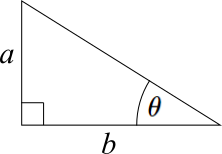It is easy to see, if a right angle triangle is drawn, in which, for an angle $\theta$ in the right angled triangle, if $\tan{\theta}=\frac ab$, then $\sin{\theta}=\sin{\arctan{\frac ab}}=\dfrac{a}{\sqrt{a^2+b^2}}$. Applying this logic, $$\sin{ \arctan{\dfrac{7a_xb_y}{5a_xb_x-2a_x^2+3b_x^2+3b_y^2}}}=\frac{7a_xb_y}{\sqrt{\left(7a_xb_y\right)^2+\left(5a_xb_x-2a_x^2+3b_x^2+3b_y^2\right)^2}}$$
which, again after a lot of algebraic manipulation, comes to $$\sin{F\hat{H}G}=\frac{7a_xb_y}{\sqrt{4a_x^4-20b_xa_x^3+\left(13b_x^2+37b_y^2\right)a_x^2+30b_x\left(b_x^2+b_y\right)^2a_x+9\left(b_x^2+b_y^2\right)^2}}$$
which is in fact equal to $$\sin{F\hat{H}G}=\frac{7a_xb_y}k$$

Now, we can find the area $A$ of the green triangle $$A=\frac{(HF)(HG)}2\sin{F\hat{H}G}\\ \Rightarrow A=\frac{\frac k{98}\times 7a_xb_y}k\\ \Rightarrow A=\frac{a_xb_y}{14}$$
The area $A'$ of the largest triangle is $\dfrac{a_xb_y}2$

Therefore, the answer to the question is $$\dfrac{A}{A'}=\dfrac{\frac{a_xb_y}{14}}{\frac{a_xb_y}2}=\frac17$$